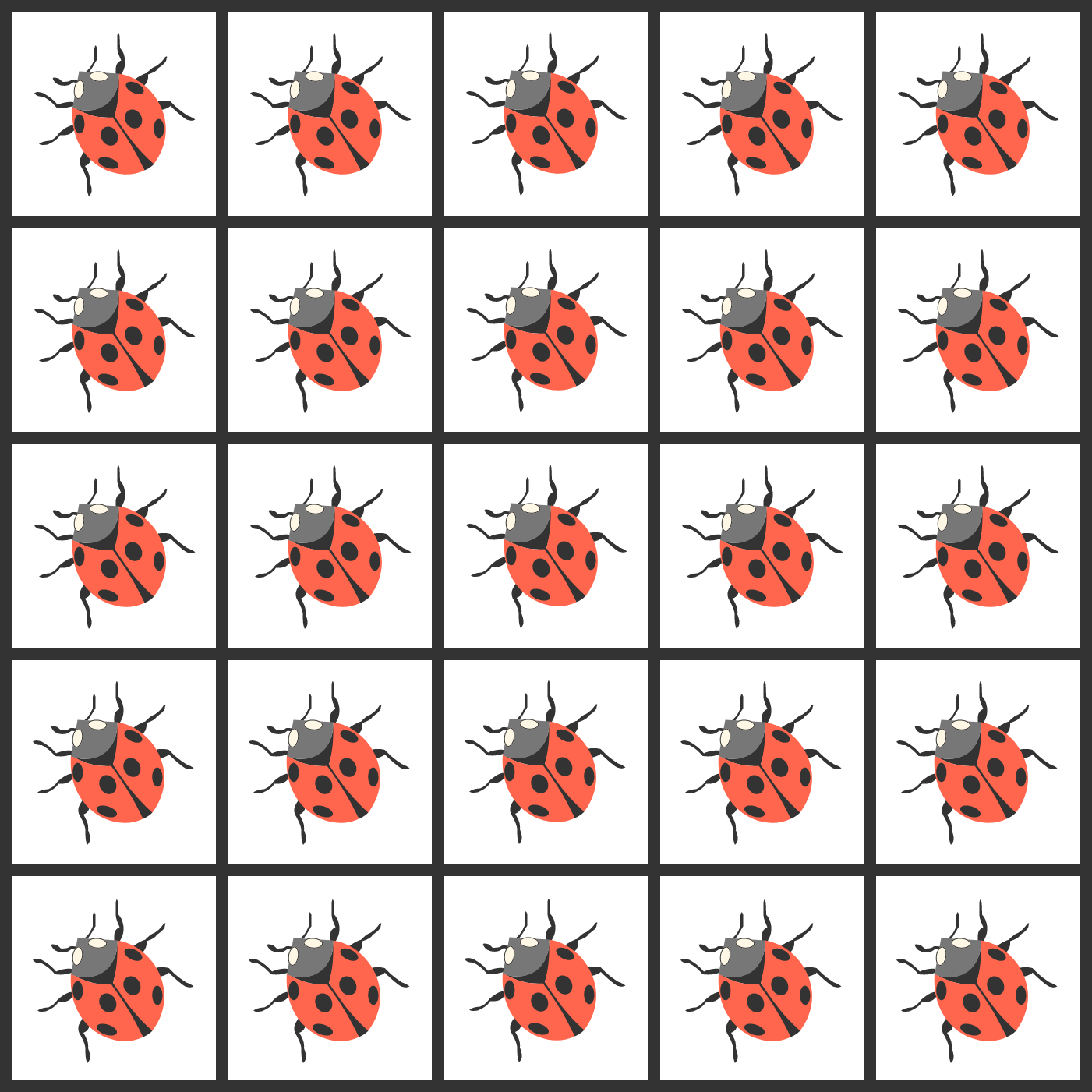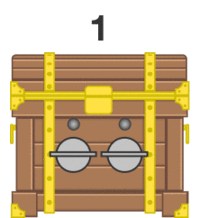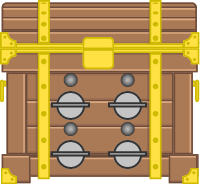# Problems of the Week

Contribute a problem

# 2017-08-14 Basic

The diagram below shows two identical, large squares divided into smaller squares.

Which area is larger, blue or green?A swing is in motion with a girl sitting on it. If the girl stands up on the swing when the swing is at the lowest point, what happens to the time period of the swing?If $a + b = 5 + 5$ and $\displaystyle \frac 1a + \frac 1b = \frac15 + \frac15,$ is it necessarily true that $a\times b = 5\times5?$

There is exactly one ladybug in each cell of a $5\times 5$ square, as shown in the figure. Then, each ladybug simultaneously flies into a randomly selected, adjacent square that shares an edge.

Is it possible that, after all the ladybugs have moved, there is still only one ladybug in each cell?Peggy is a treasure hunter trying to open two treasure boxes. One box has 2 switches and the other has 4 switches. All of the switches are initially turned off. To open a treasure box,

• she must only change one switch at a time;
• she needs to register every possible combination exactly once.

Peggy successfully opens the box with 2 switches in the following manner:The sequence in which Peggy toggles the switches

Can Peggy open the box with 4 switches?